# SEPC: Using 3D convolution to extract scale-invariant features from FPN, a rising point artifact | CVPR 2020

Posted May 26, 20205 min read

The paper proposes that PConv is a 3D convolution of the feature pyramid, which is combined with a specific iBN for regularization, which can effectively merge the internal relationship between the scales. In addition, the paper proposes SEPC, which uses deformable convolution to adapt to the corresponding features between the actual features. Regularity to maintain a balanced scale. PConv and SEPC have significantly improved the detection algorithm of SOTA, and did not bring too much extra calculation

Source:Xiaofei's algorithm engineering notes

Paper:Scale-Equalizing Pyramid Convolution for Object Detection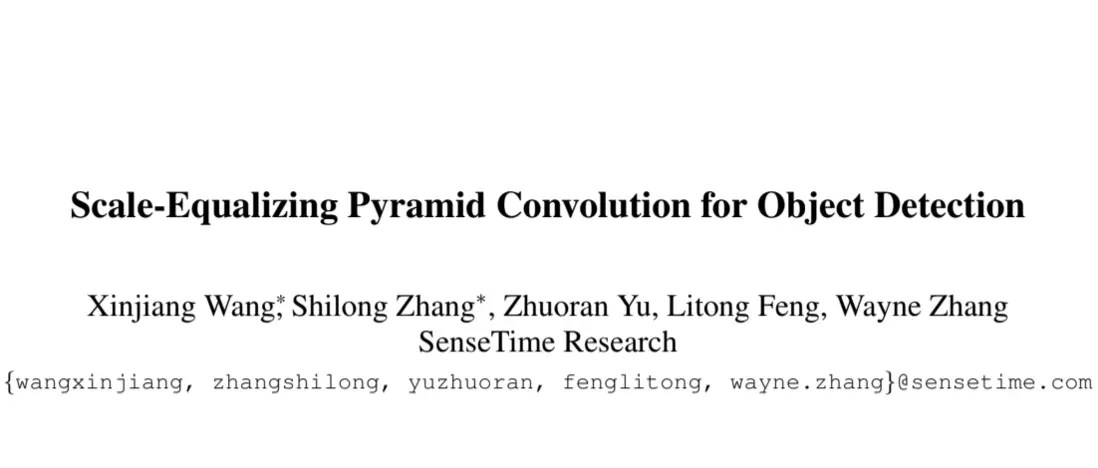# IntroductionFeature pyramid is an important means to solve the problem of object scale, but feature maps of different levels actually have a large semantic gap. In order to eliminate these semantic gaps, many studies focus on how to enhance the fusion of features, but most of these studies directly add and subtract feature maps, and do not consider the intrinsic attributes of the feature pyramid well. Inspired by scale space theory(multi-scale feature point extraction), the paper proposes PConv(pyramid convolution), which uses 3-D convolution to correlate similar feature maps and mine interactions between scales. Considering that the features of the feature pyramid vary greatly between layers and the correspondence between the points in the layers is irregular, the paper proposes SEPC(scale-equalizing pyramid convolution) to deform the convolution of the high-level features of the feature pyramid, which can adapt to the actual scale changes To maintain a balanced scale between layers.
The main contributions of the paper are as follows:

• Proposed lightweight pyramidal convolution PConv, 3-D convolution of feature pyramid to mine the correlation of inner scale.
• Proposed scale-equilibrium pyramid convolution SEPC to reduce the difference between feature pyramid and Gaussian pyramid(the paper proves that PConv has scale invariance on Gaussian pyramid).
• This module can improve the performance of SOTA single-stage target detection algorithm, and almost does not affect the speed of inference.

# Pyramid convolutionPConv(pyramid convolution) is actually a 3-D convolution that spans scale and spatial dimensions. As shown in Figure 4a, PConv can be expressed as N different 2-D convolutions.However, the feature map sizes of different pyramid levels are different. In order to accommodate different sizes, PConv uses different stride when processing different feature maps. The paper samples \$N = 3 \$, and the stride of the first convolution kernel is 2. The stride of the smallest convolution kernel is 0.5.PConv can be expressed as formula 1, \$w \ _1 \$, \$w \ _0 \$and \$w \ _ {-1} \$are three independent 2-D convolution kernels, and \$x \$is the input feature map, \$\ * \ _ {s2} \$represents a convolution kernel with stride 2.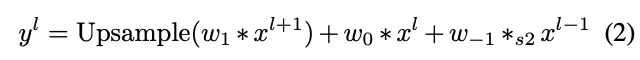The convolution kernel with stride of 0.5 first upsamples the feature map bilinearly twice, and then uses the convolution kernel with stride of 1 for processing. PConv also uses zero-padding. For the bottom and top pyramid levels, only two of Equation 2 are required. The calculation of PConv is about 1.5 times the original FPN.

### Pipeline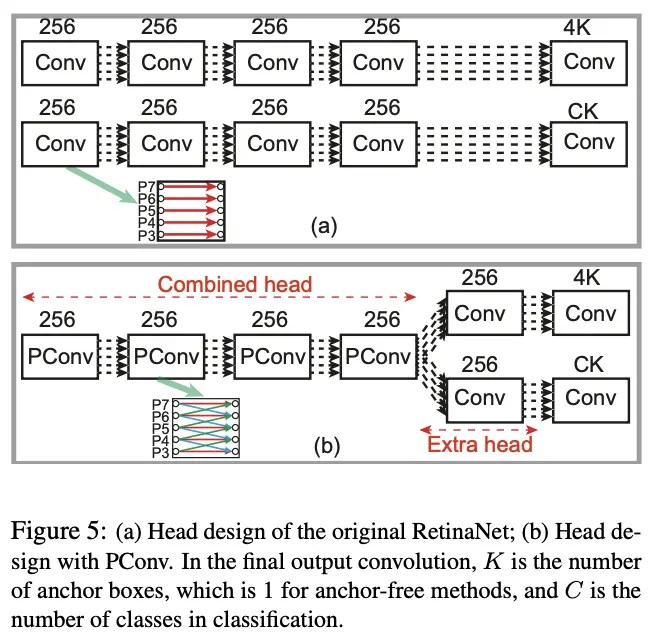As shown in Figure 5a, RetinaNet can be regarded as a PConv of \$N = 1 \$. Replace 4 Conv heads with a PCNv head of \$N = 3 \$. Stacked PConv can effectively improve the correlation gradually, and will not bring Too much extra calculation. But in order to reduce the amount of calculation as much as possible, you can choose to classify and locate the branch to share 4 layers of PConv first, and then add an additional layer of common convolutional layers, as shown in Figure 5b. This design requires even less calculation than the original RetinaNet , The specific calculation can see the original Appendix 1.

### Integrated batch normalization(BN) in the head

PConv uses a shared BN layer to count all feature maps in the feature pyramid instead of single map statistics. Since the statistics come from all the feature maps in pyramid, the variance will become smaller. In this way, even with a small batch size, the BN layer can be trained well(the variance is stable).

# Scale-equalizing pyramid convolutionPConv uses a fixed convolution kernel size for different levels. On the Gaussian pyramid(the degree of blur is not serious and the Gaussian kernel is close to the scale of the feature map), PConv can extract features with constant scale. For specific proof, see the original Appendix 3.
However, in practice, due to the existence of multi-layer convolution and nonlinear operations, the blur degree of the feature pyramid is much more serious than that of the Gaussian pyramid(the scale of the feature may not be proportional to the size of the feature map), and the use of a fixed convolution kernel size is very It is difficult to extract features with constant scale. To this end, the paper proposes SEPC(scale-equalizing pyramid convolution), which uses deformable convolution for the high-level features except the bottom layer, and predicts an offset separately, which can adapt the blur degree of each layer and maintain the scale balance between feature maps. Thereby extracting features with constant scale.
SEPC mainly has the following benefits:

• The adaptability of deformable convolution can deal with the larger level of blur between feature pyramids.
• Eliminate the difference between the feature pyramid and the Gaussian pyramid(the paper proves that PConv can extract the features of the Gaussian pyramid that have the same features).
• Since the calculation of the convolution of the high-level features is reduced by 4 times compared to the low-level(area reduction), adding deformable convolution to the high-level brings only a small amount of additional calculation.

SEPC is divided into two versions. SEPC-full adds SEPC to the Combined head and Extra head of Figure 5b, while SEPC-lite only adds SEPC to the Extra head.

# Experiments

### Single-stage object detectors### Effect of each component### Comparison of different BN implementations in the headThe output of the BN layer \$y = \ gamma \ frac {x-\ mu} {\ sigma} + \ beta \$, \$\ gamma \$and \$\ beta \$are parameters, \$\ mu \$And \$\ sigma \$is the statistical result, the comparison of the three BNs in Figure 7, where Integrated BN(iBN) is the shared BN proposed in the paper, all parameters and statistical sharing

### Comparison with other feature fusion modules### Comparison with state-of-the-art object detectors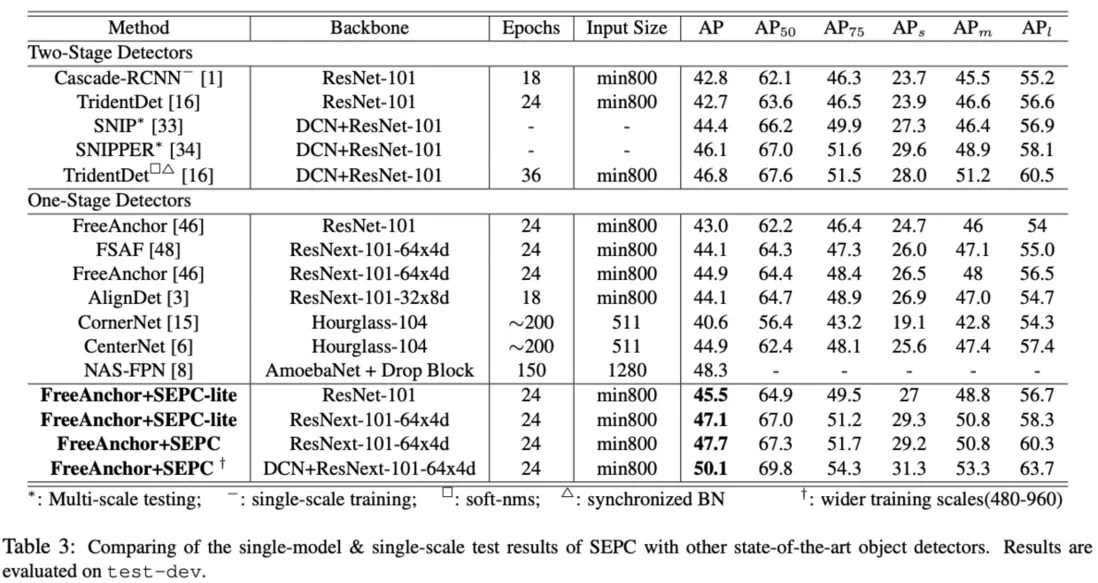### Extension to two-stage object detectors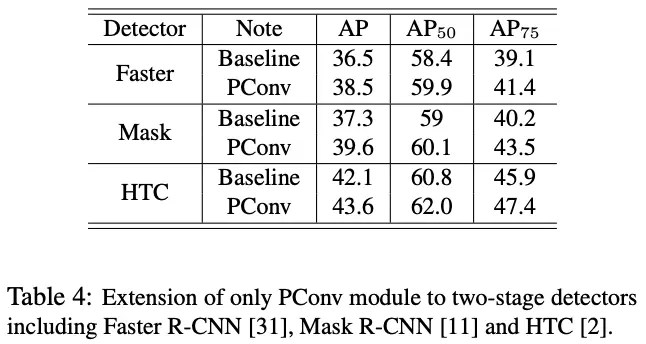# CONCLUSION

The paper proposes that PConv is a 3D convolution of the feature pyramid, which is combined with a specific iBN for regularization, which can effectively integrate the internal relationship between the scales. In addition, the paper proposes SEPC, which uses deformable convolution to adapt to the irregularities between the corresponding features Sex and maintain a balanced scale. PConv and SEPC have significantly improved the SOTA detection algorithm, and did not bring too much extra calculation.